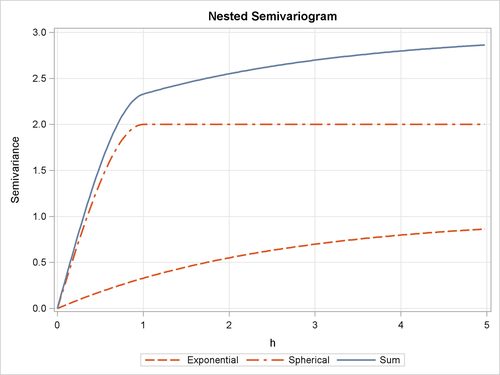Theoretical Semivariogram Models

Consider a stochastic spatial process represented by the stationary spatial random field (SRF) {} (Christakos, 1992). The VARIOGRAM procedure computes the empirical (also known as sample or experimental) semivariance of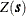. Prediction of the spatial processat unsampled locations by techniques such as ordinary kriging requires a theoretical semivariogram or covariance.

When you use PROC VARIOGRAM and PROC KRIGE2D to perform spatial prediction, you must determine a suitable theoretical semivariogram based on the sample semivariogram. Various methods exist to fit semivariogram models, such as least squares, maximum likelihood, and robust methods (Cressie, 1993, section 2.6). You can use PROC VARIOGRAM to perform automated fitting of a semivariogram model with weighted or ordinary least squares. A different approach is manual fitting, in which a theoretical semivariogram is chosen based on visual inspection of the empirical estimate; see, for example, Hohn (1988, p. 25).

In some cases, a plot of the experimental semivariogram suggests that a single theoretical model is inadequate. Nested models, anisotropic models, and the nugget effect increase the scope of theoretical models available. All of these concepts are discussed in this section. The specification of the final theoretical model is provided by the syntax of PROC KRIGE2D.

Figure 49.5 shows the general flow of investigation. The empirical semivariogram is computed after a suitable choice is made for the LAGDISTANCE= and MAXLAGS= options in PROC VARIOGRAM, and possibly the NDIR= option or the DIRECTIONS statement for computations in more than one directions. Potential theoretical models (which can also incorporate nesting, anisotropy, and the nugget effect) are then plotted against the empirical semivariogram and evaluated. A suitable theoretical model is found by using the methodology presented in the section Examples: VARIOGRAM Procedure in the VARIOGRAM procedure.

Eight theoretical models are supported by PROC KRIGE2D: the Gaussian, exponential, Matérn, spherical, cubic, pentaspherical, sine hole effect and power models. See also the section Theoretical Semivariogram Models in the VARIOGRAM procedure. These eight model forms are now examined in more detail: the Gaussian, exponential, and Matérn forms are examined as one group; the spherical, cubic, and pentaspherical as a second group; and the remaining power and sine hole effect models are examined individually. For comparison purposes, the axes in the forms’ illustrations are kept the same across the plots, and the corresponding parameters of the different forms have the same values.

In PROC KRIGE2D the parametersand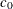for all forms correspond to the RANGE= and SCALE= options, respectively, in the MODEL statement. For all model forms, the dimension ofis the same as the dimension of the variance of the spatial process. For all forms but the power model, the dimension ofis length with same units as the distance h in the semivariance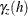. See the section The Power Semivariogram Model for more details about interpretation of the power modelparameter.

Figure 49.5: Flowchart for Semivariogram Selection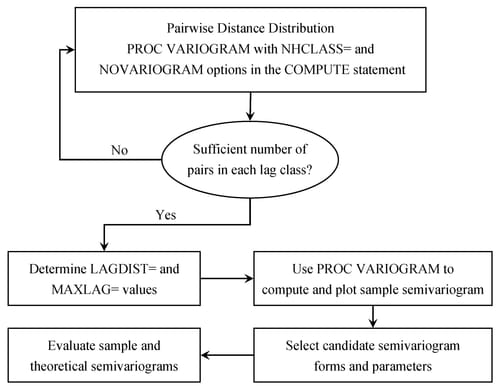The Gaussian Semivariogram Model

The form of the Gaussian model isThe shape is displayed in Figure 49.6, using rangeand scale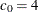.

The vertical line at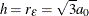shows the effective (or practical) range as defined by Deutsch and Journel (1992) or the rangedefined by Christakos (1992). The effective range is the h-value where the covariance is approximately 5% of its value at zero. Alternatively, the stationarity assumption implies that the effective range is the h value where the semivariance is approximately 5% of the sill value, as shown in Figure 49.6.

In the Gaussian model the semivarianceapproaches the sill asymptotically at.

The Exponential Semivariogram Model

The form of the exponential model is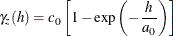The shape is displayed in Figure 49.6, using rangeand scale.

The vertical line atis the effective (or practical) range or the range(that is, the h-value where the covariance is approximately 5% of its value at zero).

As in the Gaussian model, the sill in this example is at 4.0 variance units (corresponding to) and is approached asymptotically.

The major distinguishing feature of the Gaussian and exponential forms is the shape in the neighborhood of the origin h = 0, as Figure 49.6 illustrates. In general, small lags are important in determining an appropriate theoretical form based on an empirical semivariogram.

The Matérn Semivariogram Model

The form of the Matérn model iswhereis the smoothness factor parameter. Figure 49.6 shows an example of the Matérn form, where range, scale, and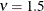.

The Matérn semivarianceis a class of semivariance models that emerge for different values of the smoothing parameter. The Matérn form reaches its sill valueasymptotically.

The Gaussian and exponential semivariances are two frequently used members of the Matérn class of semivariances. In particular, the exponential semivariance model is derived from the Matérn class of models for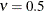. Also, when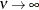then the Matérn semivariance gives the Gaussian model. In Figure 49.6 the selected value ofplaces the Matérn form in between the Gaussian and the exponential. The Matérn semivariance typically begins to look and behave as the Gaussian for values of.

Figure 49.6: Gaussian, Exponential, and Matérn Semivariograms with Parameters,, and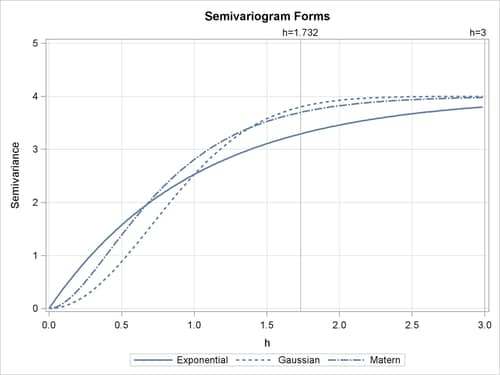The Spherical Semivariogram Model

The form of the spherical model is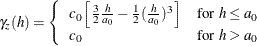The shape is displayed in Figure 49.7, using rangeand scale.

The vertical line at h = 1 shows the rangeof the model.

In the case of the spherical model,actually reaches the sill value at, unlike the Gaussian and exponential types where the sill is a horizontal asymptote.

The Cubic Semivariogram Model

The form of the cubic model is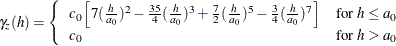The cubic form shape is displayed in Figure 49.7, using rangeand scale.

The vertical line at h = 1 shows the rangeof the model.

Similarly to the spherical model, the cubic model,reaches the sill value atand maintains this value after a distance h equal to the model range.

The Pentaspherical Semivariogram Model

The form of the pentaspherical model isThe pentaspherical form shape is displayed in Figure 49.7, using rangeand scale.

The vertical line at h = 1 shows the rangeof the model.

The pentaspherical semivariance behaves like the spherical and cubic semivariances, in thatincreases with distance until it reaches the sill valueat the distance h equal to the model range.

Figure 49.7 accents the differences in the behavior of the featured semivariances. Specifically, the cubic and pentaspherical forms reach the sill value faster than the spherical form. Also, the spherical and pentaspherical forms exhibit a more linear behavior at distances close to the origin h = 0.

Figure 49.7: Spherical, Cubic, and Pentaspherical Semivariograms with ParametersandThe Sine Hole Effect Semivariogram Model

The form of the sine hole effect model isFigure 49.8 shows an example of the sine hole effect form, where rangeand scale.

The vertical line at h = 1 shows the rangeof the model.

The sine hole effect semivarianceincreases with distance. It has the distinct characteristic that it reaches the sill at a distance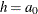equal to the model range and then it oscillates around the sill value with a decreasing amplitude as it moves to higher values of h.

The Power Semivariogram Model

The form of the power model is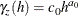For this model, the parameteris known as the power exponent. This is a dimensionless quantity which must range within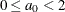so that the power model is a permissible semivariance model.

The KRIGE2D procedure enables you to specify power exponent values that are outside this range when you also explicitly specify the POWNOBOUND option in the MODEL statement. However, parameter values equal to or greater than 2 can result in singular covariance matrices or negative prediction errors.

For the special case ofthe form yields a straight line. In this case the power model reduces to the linear model. The parameterdesignates the slope of the power form and has dimensions of the variance as in the other models.

The power model has no sill; this differentiates it from the rest of the models presented earlier. Spatial correlation that is described by a power model indicates that the stochastic process variance increases constantly with distance. The shape of the power model with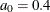andis displayed in Figure 49.8.

Figure 49.8: Sine Hole Effect Semivariogram with Rangeand Scale, and Power Semivariogram with Exponentand Slope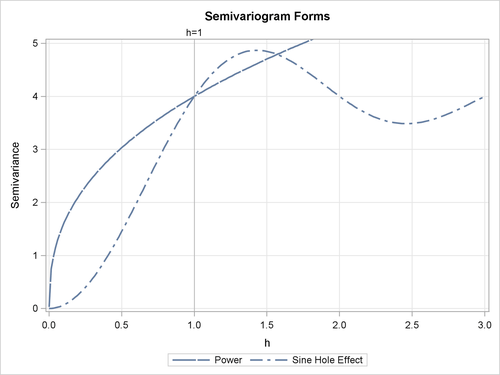For comparison purposes, Figure 49.9 displays all eight semivariance forms that you can use with PROC KRIGE2D. The figure displays a composition of the different forms with the parameter values selected earlier throughout this section. Depending on the empirical semivariogram, these models provide you with flexibility to select an appropriate theoretical semivariance model for prediction.

Figure 49.9: Semivariogram Forms Used in PROC KRIGE2D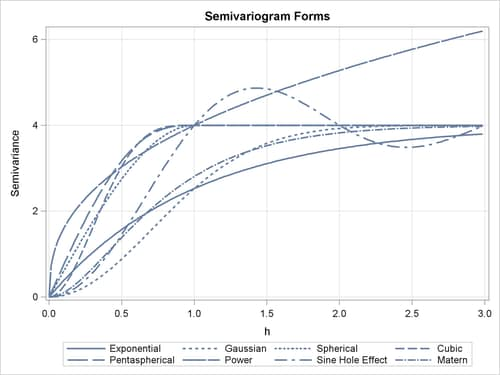Nested Models

For a given set of spatial data, a plot of an experimental semivariogram might not seem to fit any of the individual theoretical models. In such a case, you might obtain a more accurate fit if you consider your covariance model to be the sum of two or more covariance structures. Such covariance models are called nested models. Nesting is common in geologic applications where correlations can exist at different length scales. At small lag distances h, the smaller scale correlations dominate, while the large scale correlations dominate at larger lag distances.

Nested models are permissible covariances if they are the sum of permissible models. Therefore, you can include in a sum any combination of the models presented in the preceding subsections and produce permissible covariance models. As an illustration, consider two semivariogram models: an exponential and a spherical,and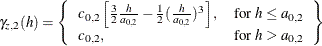with, and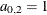. If both of these correlation structures are present in a spatial process {}, then the semivariance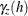of this process can be expressed as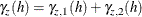In this case, the experimental semivariogramfor the processresembles the semivariogram of the sum of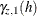and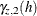. This is illustrated in Figure 49.10.

The sum ofandin Figure 49.10 does not resemble any single theoretical semivariogram; however, its shape at h = 1 is similar to a spherical form. The asymptotic approach to a sill at three variance units, along with the shape around h = 0, indicates an exponential structure. The sill valueof the sum is the sum of the individual sills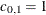and. In general, a nested model has a sill equal to the sum of the sills of its nested structures plus the nugget effect, if present.

See Hohn (1988, p. 38ff) for further examples of nested correlation structures.

Figure 49.10: Sum of Exponential and Spherical Structures at Different Scales